# Using the `eesim` package

## Overview of package

This package allows you to simulate time series of environmental health data and perform simulation-based power analyses and other measures of model performance. The package includes four main parts:

1. Generation of exposure data (simulated or from real data);
2. Generation of simulated outcome data;
3. Fitting models to generated data; and
4. Evaluating model performance on generated data.

The user has the option to customize different aspects of the simulation at each of these steps.

The package creates simulated time series data that are relevant for environmental epidemiology studies of ambient exposures (e.g., studies of acute mortality risks associated with daily air pollution concentration, daily temperature, or occurance of a community-wide extreme event like a heat wave). Simulated environmental datasets like those created by the package can be used in to assess the performance of statistical models meant to estimate the association between exposure level and outcome risk, to estimate power for a planned study, and to develop a better understanding of the data generating processes behind observed environmental datasets. Such time series are often characterized by both seasonal and long-term trends in both the exposure of interest and the outcome. For example, the following plot shows time series of daily ozone concentration (in parts per billion [ppb]) and cardiovascular deaths in Chicago, IL (1996–2000), with smoothed lines overlaid on the raw data to show patterns over time.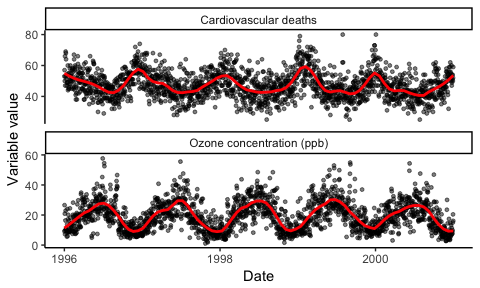## Basic example of using the package

The main function of this package is the `eesim` function. You can use the `eesim` function to conduct all four steps of the simulation process at once (generate exposure data, generate outcome data, fit models to simulated data, and evaluate model performance).

The `eesim` function requires inputs on:

• `n`: The desired number of observations per simulated dataset (for a daily time series, this is the desired number of days in the simulated dataset)
• `n_reps`: The desired number of simulated datasets
• `exposure_type`: Whether the exposure is binary (e.g., occurence of an extreme event like a heat wave or wildfire) or continuous (e.g., concentration of a pollutant)
• `rr`: The relative rate of the outcome associated with the exposure. For a binary exposure, this is the relative rate associated with the exposure compared to a similar day without the exposure. For a continuous exposure, this is the relative rate associated with a one-unit increase in the exposure.
• `model`: The model to be used to estimate the association between exposure and outcome in the simulated datasets, either to estimate power of a planned analysis or to otherwise evaluate the performance (e.g., coverage, bias) of a model on the simulated datasets.

A number of optional inputs can also be specified, including arguments to adjust the shape of seasonal or long-term trends in the exposure or outcome data or custom arguments to use at different steps of the data generation.

The function returns a list with three elements. The first element is a list with all the simulated datasets. The second element gives simulation-specific results for each simulated dataset: the estimated effect, standard error, t- and p-values, and upper and lower 95% confidence bounds when a model was applied to each of the simulated datasets. The third element gives some measures of model assessment, assessed over all simulations, including the mean beta and relative risk estimates across simulations.

For example, in the observed data from Chicago, IL, shown in the plots above, daily ozone concentrations have a mean of about 20 ppb and standard deviation of about 7 ppb after removing seasonal and long-term trends. The average number of cardiovascular deaths per day is around 50. Here is the code, and a plot of the resulting data, for generating a dataset with similar characteristics for use in a power analysis or to evaluate model performance (later in the vignette, we will show how to use customization to further improve the simulation of data for this example, including avoiding negative values of ozone concentration in simulated data):

``````sim_chicago <- create_sims(n_reps = 1, n = 365 * 5, central = 20, sd = 7,
exposure_type = "continuous", exposure_trend = "cos1",
exposure_amp = -.6, average_outcome = 50,
outcome_trend = "cos1", outcome_amp = 0.2,
rr = 1.0005, start.date = "1996-01-01")
``````##         date         x outcome
## 1 1996-01-01 11.528649      67
## 2 1996-01-02 -4.835049      59
## 3 1996-01-03  4.357889      49
## 4 1996-01-04  6.986876      51
## 5 1996-01-05  8.455094      44
## 6 1996-01-06  5.309139      53``````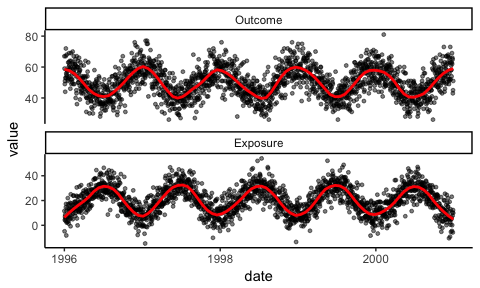This simulated data can also be visualized using the `calendar_plot` function that comes with the package:

``````a <- calendar_plot(sim_chicago[] %>% select(date, outcome), type = "continuous",
legend_name = "Outcome") +
ggtitle("Outcome")
b <- calendar_plot(sim_chicago[] %>% select(date, x), type = "continuous") +
ggtitle("Exposure")
grid.arrange(a, b, ncol = 1)``````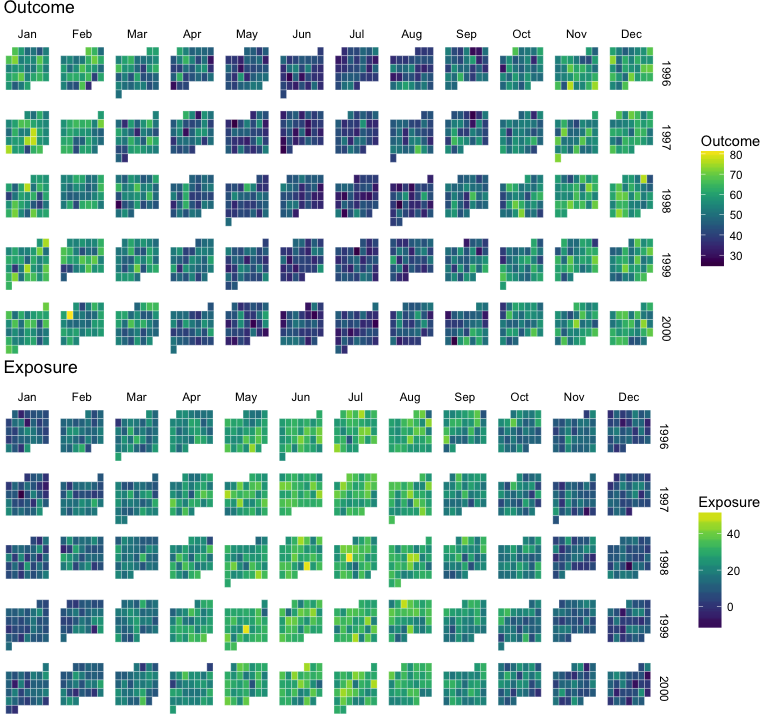You can use the `eesim` function to generate multiple similar simulated datasets and investigate the performance of a specified model in estimating the association between ozone concentration and the risk of cardiovascular death in 20 simulated datasets. You must write a function with the code to fit the model you desire to fit to the simulated data (more details for writing this function are provided later in the vignette), and then you can use the `eesim` function to generate lots of simulated datasets, fit that model, and assess its performance using a call like:

``````ex_sim <- eesim(n_reps = 100, n = 365 * 5, central = 20, sd = 7,
exposure_type = "continuous", exposure_trend = "cos1",
exposure_amp = -.6, average_outcome = 50,
outcome_trend = "cos1", outcome_amp = 0.2,
rr = 1.2, start.date = "1996-01-01",
custom_model = spline_mod, custom_model_args = list(df_year = 7))``````

The `eesim` function returns a list with three elements:

``names(ex_sim)``
``##  "simulated_datasets"  "indiv_performance"   "overall_performance"``

The first element of the returned object is a list with all of the simulated datasets. For example, you can create a calendar plot of exposure in the first simulated dataset using the call:

``calendar_plot(ex_sim[["simulated_datasets"]][] %>% select(date, x), type = "continuous")``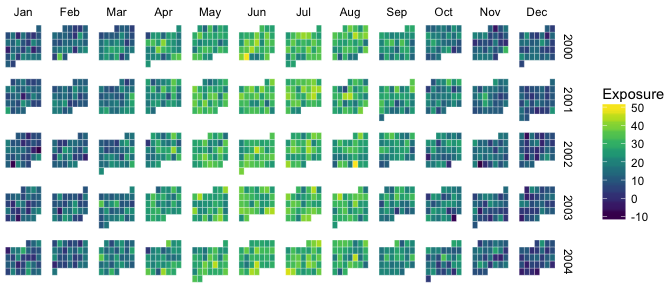The second element of the returned object gives the results of fitting the model to each of the simulated datasets. It can be used to explore the behavior of individual simulations:

``head(ex_sim[["indiv_performance"]])``
``````##    Estimate    Std.Error  t.value p.value  lower_ci  upper_ci
## 1 0.1822613 0.0005431742 335.5485       0 0.1811967 0.1833259
## 2 0.1818704 0.0004819506 377.3632       0 0.1809258 0.1828150
## 3 0.1813407 0.0005127487 353.6638       0 0.1803357 0.1823456
## 4 0.1824330 0.0005117458 356.4915       0 0.1814300 0.1834360
## 5 0.1835011 0.0005139293 357.0551       0 0.1824938 0.1845084
## 6 0.1819087 0.0005127441 354.7748       0 0.1809037 0.1829137``````

After running the simulation, you can look at the relative risk point estimate and 95% confidence interval from each of the 100 simulations, as well as which 95% confidence intervals include the true relative rate, using the `coverage_plot` function that comes with the package:

``coverage_plot(ex_sim[["indiv_performance"]], true_param = 1.2)``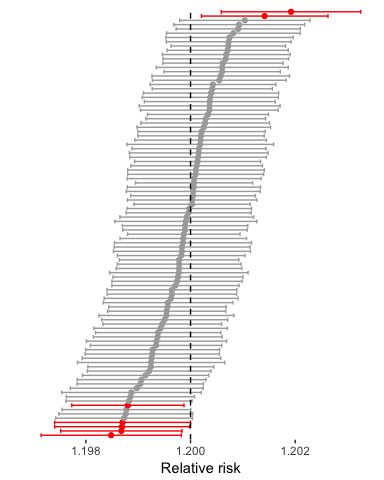The third element of the list returned by a call to `eesim` gives the following overall summaries of model performance across all simulations:

Variable Description
`beta_hat` Mean estimate: The mean of the estimated log relative rate over all simulations.
`rr_hat` Mean estimated relative rate: The mean of the estimated relative rate over all simulations.
`var_across_betas` Variance across estimates: Variance of the point estimates (estimated log relative risk) over all simulations.
`mean_beta_var` Mean variance of estimate: The mean of the variances of the estimated effect (estimated log relative risk) across all simulations.
`percent_bias` Relative bias: Difference between the estimated log relative risk and true log relative risk as a proportion of the true log relative risk.
`coverage` 95% confidence inverval coverage: Percent of simulations for which the 95% confidence interval estimate of log relative risk includes the true value of log relative risk.
`power` Power: Percent of simulations for which the null hypothesis that the log relative risk equals zero is rejected based on a p-value of 0.05.

For example, here are the overall results for the simulation fit above:

``ex_sim[["overall_performance"]]``
``````##    beta_hat    rr_hat var_across_betas mean_beta_var percent_bias coverage
## 1 0.1822211 0.1822211     3.011244e-07  2.780242e-07   0.05510361     0.93
##   power
## 1     1``````

In later sections of this vignette, we will show how to customize steps in the generation of the simulated data to further improve this example simulation.

As another basic example, here is a plot of the dates of extreme heat days (defined as a day with temperature at or above the 98 percentile temperature in Chicago between 1987 and 2000) in the observed Chicago dataset (points are jittered along the y-axis to limit overlapping):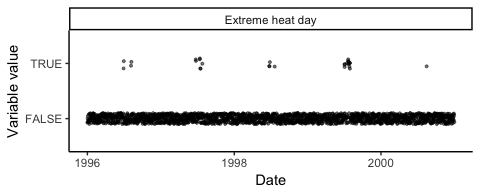In this observed data, there is (unsurprisingly) a strong seasonal trend in this binary exposure of extreme heat days. The percent of days that are extreme heat days is 0% for all months expect June (about 5% of days in observed data were extreme heat days), July (about 12% of days), and August (about 2% of days). Similar exposure time series can be simulated with the call:

``````sim_chicago2 <- create_sims(n_reps = 1, n = 365 * 5, sd = 1,
central = c(0, 0, 0, 0, 0, 0.05, 0.12, 0.02, 0, 0, 0, 0),
exposure_type = "binary", exposure_trend = "monthly",
exposure_amp = -.6, average_outcome = 50,
outcome_trend = "cos1", outcome_amp = 0.2,
rr = 1.05, start.date = "1996-01-01")``````

Here is an example of the simulated exposure data: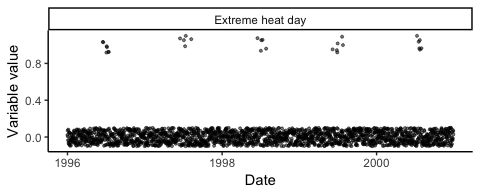Again, both the observed and simulated exposure data can also be plotted using the `calendar_plot` function:

``````a <- chicagoNMMAPS %>%
mutate(temp = temp >= quantile(temp, probs = 0.98)) %>%
tbl_df() %>%
filter(year >= 1996) %>%
select(date, temp) %>%
calendar_plot(type = "discrete", labels = c("Extreme heat day", "Other day")) +
ggtitle("Observed exposure data")
b <- sim_chicago2[] %>%
select(date, x) %>%
calendar_plot(type = "discrete", labels = c("Extreme heat day", "Other day")) +
ggtitle("Simulated exposure data")
grid.arrange(a, b, ncol = 1)``````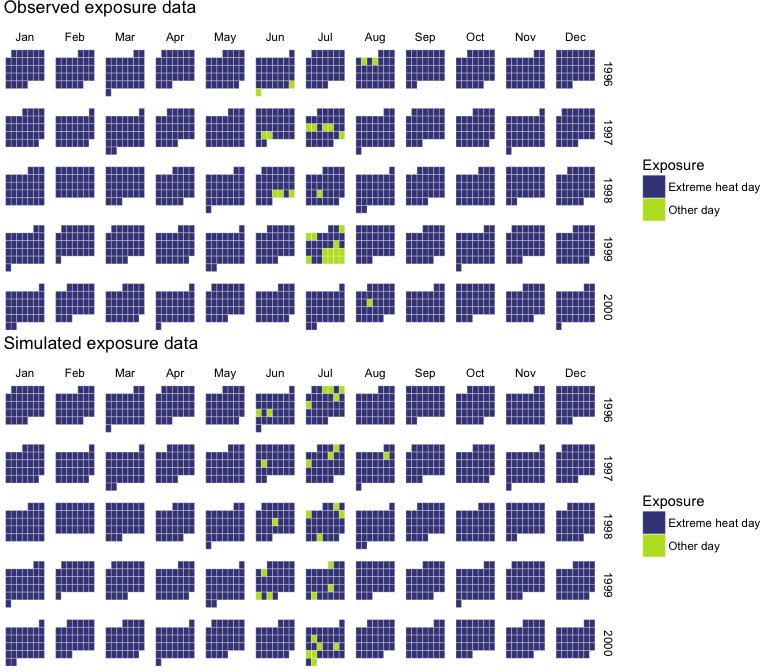The comparison of the observed and simulated data in this case suggests some clustering in the observed data that is not evident in the simulated data, suggesting that the probability of exposure may be higher on a day near other extreme heat days.

The `eesim` function can be used to assess the performance of a GLM in estimating relative risk of cardiovascular mortality for extreme heat days compared to other days using:

``````ex_sim2 <- eesim(n_reps = 100, n = 365 * 5,
central = c(0, 0, 0, 0, 0, 0.05, 0.12, 0.02, 0, 0, 0, 0),
exposure_type = "binary", exposure_trend = "monthly",
exposure_amp = -.6, average_outcome = 50,
outcome_trend = "cos1", outcome_amp = 0.2,
rr = 1.05, start.date = "1996-01-01",
custom_model = spline_mod, custom_model_args = list(df_year = 7))``````
``````## This function may take a minute or two to run, especially if you
## are creating lots of replications (`n_reps`).``````

As before, a plot of CI coverage can be created with `coverage_plot`:

``coverage_plot(ex_sim2[["indiv_performance"]], true_param = 1.05)``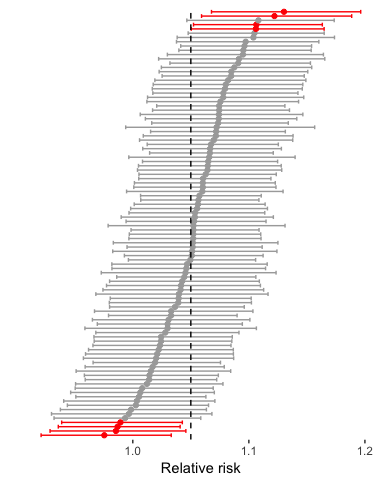Here are the overall estimates in this case for model performance:

``ex_sim2[["overall_performance"]]``
``````##     beta_hat     rr_hat var_across_betas mean_beta_var percent_bias
## 1 0.04996982 0.04996982     0.0009712768  0.0009160301    -2.417805
##   coverage power
## 1     0.92  0.43``````

In this case, the expected power is low.

The `power_calc` function in the package allows you to extend on this simulation functionality to create power curves for an analysis given an anticipated underlying process of data generation. This function will create simulations for several different values of number of days in the study (`n`), average daily outcome counts (`average_outcome`), or expected association between exposure and outcome (`rr`).

For example, the following call generates a power curve that explores how expected power changes with an increasing number of days for the heat wave analysis example just presented (as a warning, this call takes a few minutes to run, since it’s simulating many datasets):

``````ex_power_calc <- power_calc(varying = "n", values = floor(365.25 * seq(1, 20, by = 5)),
n_reps = 100, rr = 1.05,
central = c(0, 0, 0, 0, 0, 0.05, 0.12, 0.02, 0, 0, 0, 0),
exposure_type = "binary", exposure_trend = "monthly",
exposure_amp = -.6, average_outcome = 50,
outcome_trend = "cos1", outcome_amp = 0.2,
custom_model = spline_mod, custom_model_args = list(df_year = 7),
plot = FALSE)``````
``````## This function may take a minute or two to run, especially with
## lots of replications (`n_reps`) or options for `values`.``````
``````ex_power_calc %>%
ggplot(aes(x = values, y = power)) +
geom_line() +
ylim(0, 1) +
labs(x = "Number of days in the study", y = "Power") +
theme_bw()``````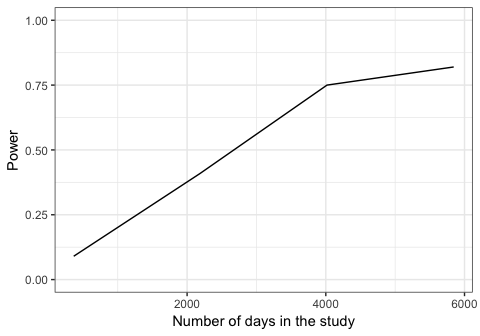## Piece-by-piece breakdown of package utility

To demonstrate how the `eesim` function works, here is a breakdown of each of the four main parts: generating exposure data, generating outcome data, fitting models, and evaluating models. The helper functions used for each step are described in detail in this section.

### Generating exposure data

The first task of the package is generating exposure data. This can be done with the `sim_exposure` function. In this function, the user can specify whether he or she would like to generate exposure data that is binary or continuous (`exposure_type`). For continuous exposure data, the user must specify the mean (`central`) and standard deviation (`sd`) of the exposure data. For example, the following call simulates a dataframe of exposure data for an exposure that is normally distributed, with a mean value of 50, a standard deviation of 5, and no long-term or seasonal trends:

``````x_cont <- sim_exposure(n = 1000, central = 50, sd = 5, exposure_type = "continuous")
x_cont %>% slice(1:5)``````
``````##         date        x
## 1 2001-01-01 49.26604
## 2 2001-01-02 42.91050
## 3 2001-01-03 52.53690
## 4 2001-01-04 51.53205
## 5 2001-01-05 52.42711``````
``````ggplot(x_cont, aes(x = date, y = x)) + geom_point(alpha = 0.2) +
theme_classic()``````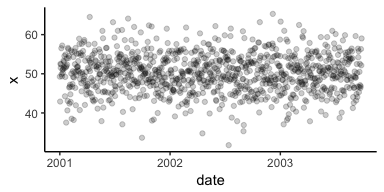You can plot a calendar plot of this simulated exposure time series using the `calendar_plot` function that comes with the package. Within this function, the type of data (“continuous” or “discrete”) must be specified:

``calendar_plot(x_cont, type = "continuous")``